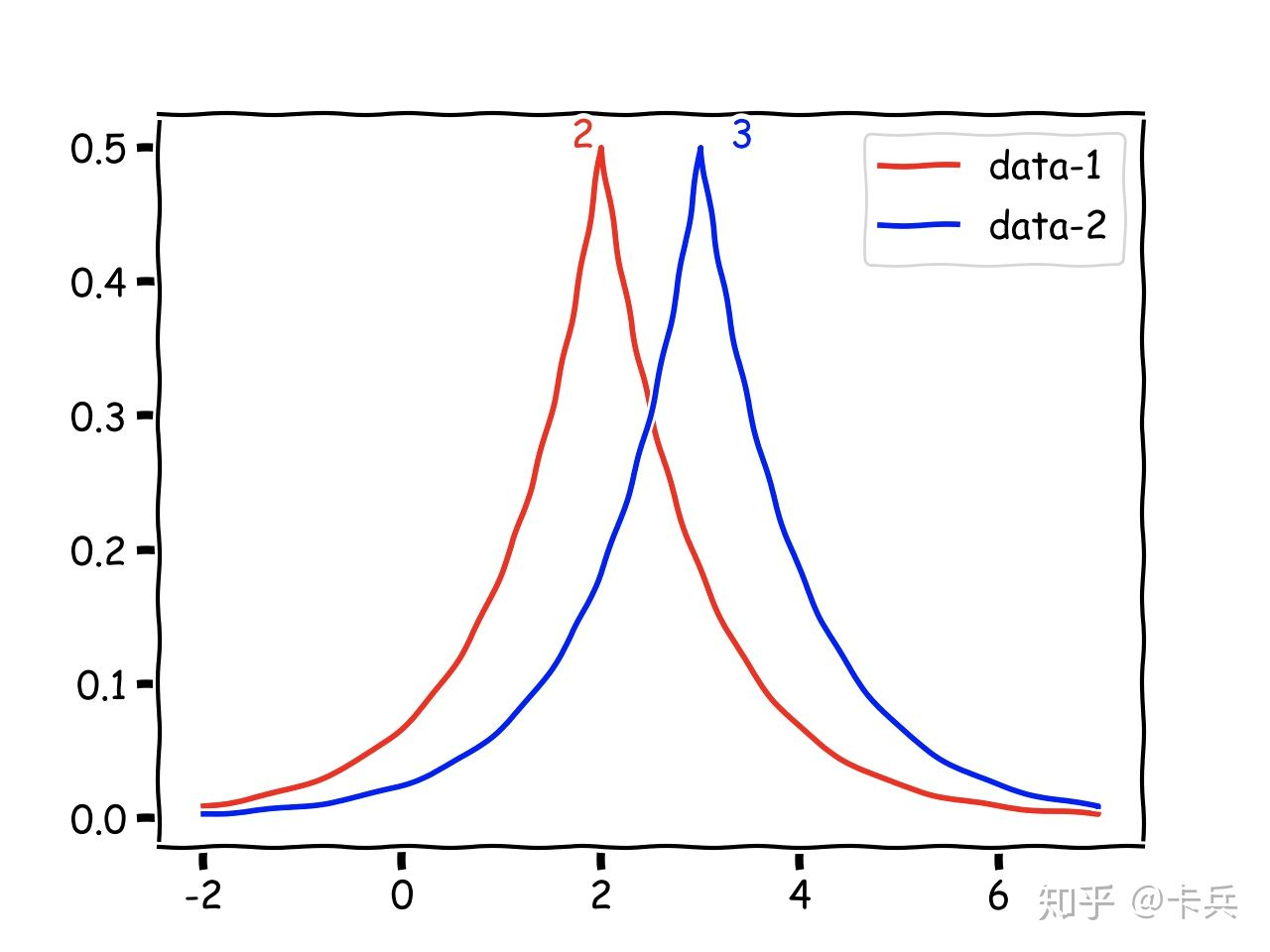什么是差分隐私

https://zhuanlan.zhihu.com/p/139114240

差分隐私顾名思义就是用来防范差分攻击的，我最早接触到差分攻击的概念是数据库课上老师介绍的。举个简单的例子，假设现在有一个婚恋数据库，2个单身8个已婚，只能查有多少人单身。刚开始的时候查询发现，2个人单身；现在张三跑去登记了自己婚姻状况，再一查，发现3个人单身。所以张三单身。联邦学习中的差分隐私

• 本地计算

客户端 $i$ 根据本地数据库 $\mathcal{D}_i$ 和接受的服务器的全局模型$w_G^t$作为本地的参数，即$w_i^t = w_G^t$，进行梯度下降策略进行本地模型训练得到$w_i^{t+1}$$t$ 表示当前round）。

• 模型扰动

每个客户端产生一个随机噪音$n$$n$ 是符合高斯分布的，使用$\overline{w_i}^{t+1} = w_i^{t+1} + n$ 扰动本地模型（这里注意$w$是一个矩阵，那么$n$就对矩阵的每一个元素产生噪音）。

• 模型聚合

服务器使用FedAVG算法聚合从客户端收到的$\overline{w_i}^{t+1}$得到新的全局模型参数$w_G^{t+1}$，也就是扰动过的模型参数。

• 模型广播

服务器将新的模型参数广播给每个客户端。

• 本地模型更新

每个客户端接受新的模型参数，重新进行本地计算。

为什么联邦学习需要差分隐私？

.Melis etal. Exploiting Unintended Feature Leakage in Collaborative Learning. In lEEE Symposium on Security & Privacy,2019.

.Hitaj et al. Deep models under theGAN: information leakage from collaborative deep learning.In ACM SIGSAC Conference on

1.基于安全多方计算的

2.基于差分隐私的

https://zhuanlan.zhihu.com/p/266134450

FL中的差分隐私噪声是如何产生的？

• 首先每个client根据自己的隐私保护要求程度，产生一个差分隐私的$\epsilon$值，$\epsilon$越大隐私保护程度越弱
• 每个client是需要裁剪（Clip）梯度，要把梯度bound在$C$$-C$之间的话，那么差分隐私的$\Delta f = \frac{2C}{n}$，n为本地sample的数量
• 根据$\Delta f$$\epsilon$的值就可以求出拉普拉斯分布或者高斯分布的参数了（高斯分布可能还需要$\delta$,这个也是client自己规定的）
• 然后从高斯分布或者拉普拉斯分布中sample出一个值加上模型参数值就可以了。

$\Delta f = \frac{2C}{n}$的推导可以看这两篇论文：

1.Federated Learning with Differential Privacy:Algorithms and Performance Analysis

2.The Value of Collaboration in Convex Machine Learning with Differential Privacy

• 每个client可能要求的$\epsilon$是不一样的。
• 每个client添加的噪音是独立的，但是不一定同分布（$\epsilon,\Delta f$不一样）。

1中4.2节的描述特别清晰。